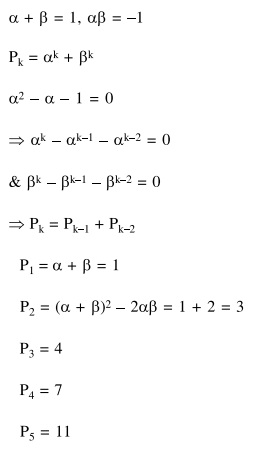# Let alpha and beta be the roots of the equation

Question:

Let $\alpha$ and $\beta$ be the roots of the equation $\mathrm{x}^{2}-\mathrm{x}-1=0$. If $\mathrm{p}_{\mathrm{k}}=(\alpha)^{\mathrm{k}}+(\beta)^{\mathrm{k}}, \mathrm{k} \geq 1$, then which one of the following statements is not true ?

1. $\left(\mathrm{p}_{1}+\mathrm{p}_{2}+\mathrm{p}_{3}+\mathrm{p}_{4}+\mathrm{p}_{5}\right)=26$

2. $\mathrm{p}_{5}=11$

3. $\mathrm{p}_{3}=\mathrm{p}_{5}-\mathrm{p}_{4}$

4. $\mathrm{p}_{5}=\mathrm{p}_{2} \cdot \mathrm{p}_{3}$

Correct Option: , 4

Solution: